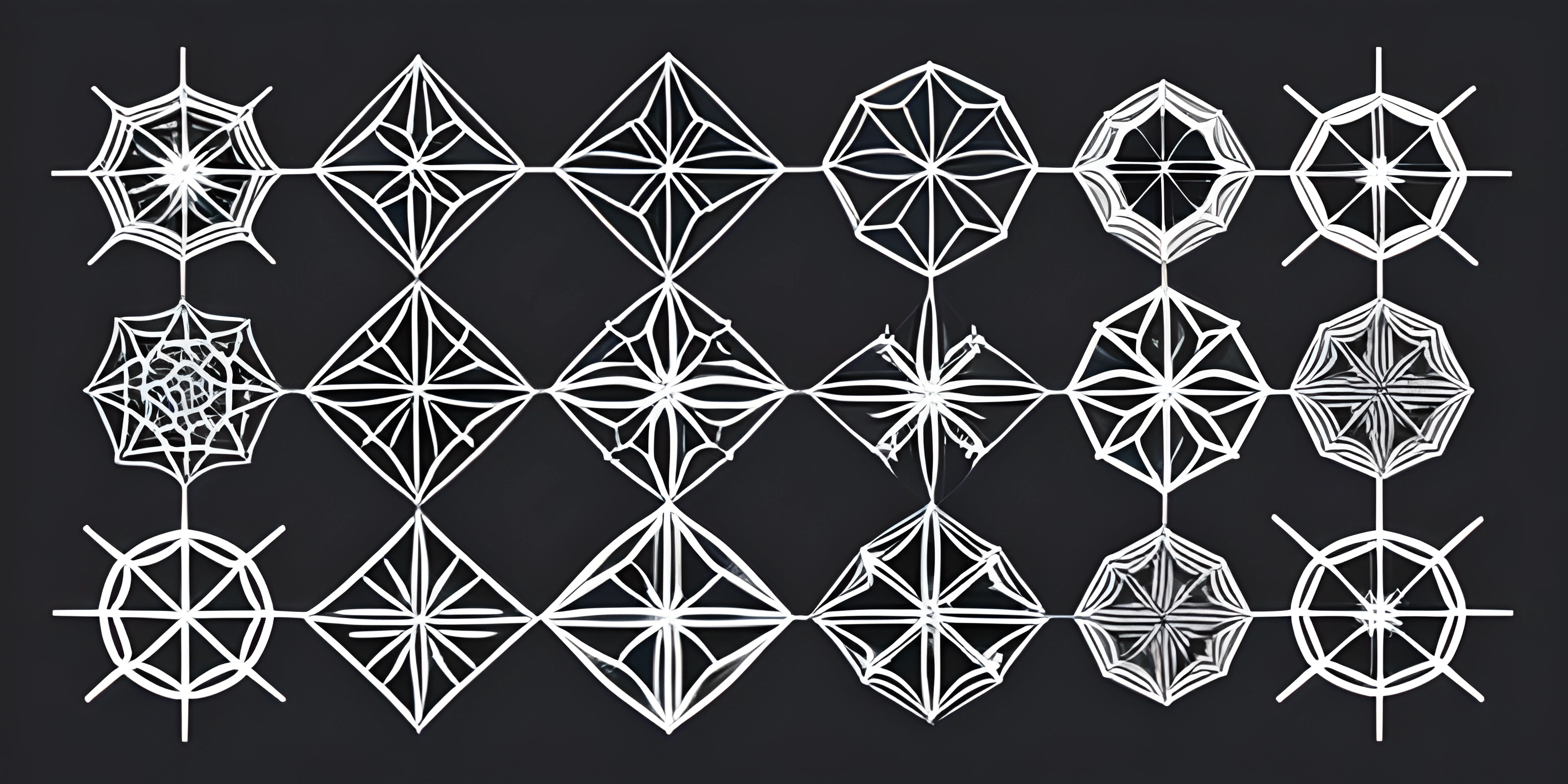# Algorithms and Data StructuresNote: this page has been created with the use of AI. Please take caution, and note that the content of this page does not necessarily reflect the opinion of Cratecode.

In the world of programming, algorithms and data structures are the foundation of efficient problem-solving. These helpful tools not only help us achieve the desired output, but also ensure that our code runs smoothly and efficiently. So let's dive into an overview of some common algorithms and data structures to give you a better understanding of their use cases and applications.

## Algorithms

Algorithms are step-by-step procedures for performing calculations or solving problems. Let's look at some popular algorithms and their use cases:

1. Bubble Sort: A simple sorting algorithm that compares adjacent elements and swaps them if they are in the wrong order.
2. Merge Sort: A divide-and-conquer sorting algorithm that recursively splits the array into halves and then merges the sorted halves together.
3. Quick Sort: Another divide-and-conquer sorting algorithm with a pivot element that partitions the array into two smaller arrays and recursively sorts them.
4. Binary Search: A search algorithm that efficiently finds a specific element in a sorted array by dividing the array into halves and narrowing down the search range.
5. Dijkstra's Algorithm: A pathfinding algorithm that finds the shortest path between nodes in a weighted graph.

## Data Structures

Data structures are ways of organizing and storing data to enable efficient access and modification. Here are a few common data structures and their use cases:

1. Array: A collection of elements, each identified by an index, that store data in a linear arrangement.
2. Linked List: A linear data structure where each element is a separate object called a node, and nodes point to the next node in the sequence.
3. Stack: A collection of elements that follows the Last In, First Out (LIFO) principle, meaning the most recently added element is the first to be removed.
4. Queue: A collection of elements that follows the First In, First Out (FIFO) principle, where the element added earliest is the first to be removed.
5. Tree: A hierarchical data structure that consists of nodes connected by edges, with a single root node and no cycles.
6. Graph: A data structure that consists of nodes and edges, representing relationships between pairs of objects.

By understanding these fundamental algorithms and data structures, you'll be well-equipped to tackle a wide variety of programming challenges. Remember, the key to efficient problem-solving lies in choosing the right algorithm and data structure for the task at hand. So keep exploring, experimenting, and refining your skills!

## FAQ

### What are some common algorithms and data structures?

There are numerous algorithms and data structures that are commonly used in computer science and programming. Some popular examples include:

• Arrays
• Stacks
• Queues
• Trees
• Graphs
• Hash Tables
• Searching Algorithms (e.g., Binary Search)
• Sorting Algorithms (e.g., Bubble Sort, Quick Sort, Merge Sort) Each of these has its unique use cases and applications in solving different types of problems efficiently.

### How do I choose the right data structure for a specific problem?

Choosing the right data structure for a specific problem depends on the nature of the problem and the operations you need to perform. Here are some factors to consider when making your decision:

• Performance requirements: Consider the complexity of the operations you'll be performing. For instance, if you need fast insertions and deletions, a linked list might be a better choice than an array.
• Memory usage: Some data structures consume more memory than others. If memory is a constraint, you might want to choose a more space-efficient data structure.
• Ease of implementation: Sometimes, a simpler data structure is easier to implement and debug, even if it's not the most efficient choice. It's important to thoroughly understand the problem you're trying to solve and weigh the trade-offs between different data structures before making a decision.

### Can you provide an example of an algorithm and its application?

Sure! Let's take a look at the Binary Search algorithm. Binary Search is an efficient searching algorithm that works on sorted lists or arrays. The algorithm repeatedly divides the list into two halves, comparing the middle element with the target value. If the middle element matches the target value, the search is successful. If the middle element is less than the target value, the algorithm continues searching in the right half of the list; otherwise, it searches in the left half. Here's a Python implementation of Binary Search:

``````def binary_search(arr, target):
left, right = 0, len(arr) - 1
while left <= right:
mid = (left + right) // 2
if arr[mid] == target:
return mid
elif arr[mid] < target:
left = mid + 1
else:
right = mid - 1
return -1``````

Binary Search is used in numerous applications, such as searching for a specific value in a database, auto-complete features in search engines, and even in computer gaming for decision-making and pathfinding.

### What are some resources for learning more about algorithms and data structures?

There are plenty of resources available to learn more about algorithms and data structures. Some popular resources include:

• Books: "Introduction to Algorithms" by Cormen, Leiserson, Rivest, and Stein, and "Data Structures and Algorithms in Python" by Goodrich, Tamassia, and Goldwasser are excellent books for learning the fundamentals.
• Online Courses: Websites like Coursera, Udacity, and edX offer various courses on algorithms and data structures, ranging from beginner to advanced levels.
• YouTube Channels: Channels like "mycodeschool" and "Tushar Roy - Coding Made Simple" provide in-depth explanations and visualizations of various algorithms and data structures.
• Competitive Programming: Participating in competitive programming contests on platforms like Codeforces, LeetCode, and HackerRank can help you practice and improve your skills in algorithms and data structures. Remember, practice makes perfect, so the more you work with algorithms and data structures, the better you'll become at using them effectively.